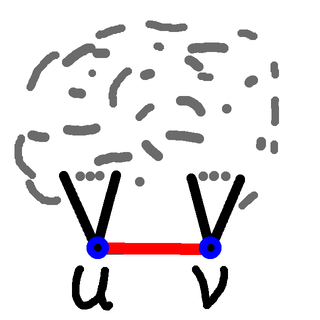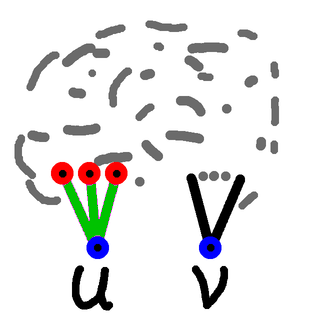# Recursion RevisitedSubscribe!My latest posts can be found here:
Previous blog posts:

# Recursion revisited ... a specific example

 This post is partly prompted by a comment on Hacker News which said: The three examples used to demonstrate recursion, towers of Hanoi, fibonacci, and binary search are not the most convincing ones. All can be solved with iteration. Others pointed out that (in principle) any recursive algorithm can be expressed as an iterative one, and vice versa, but I replied saying: Educational examples are rarely "real world", and anything "real world" is usually too complex to make the underlying principles clear ... I'm going to write a follow-up post to this with a genuine real-world (well, Pure Maths calculation) example ... perhaps you will find that more satisfying. So this is that post.
Some time ago I wrote a post on Thinking About Recursion, and more recently I wrote about a problem concerning the problem of the number of Vertices Required For Cycles. As it happens, the code for that problem is heavily recursive, and provides a great example of using recursion to solve a real problem.

So I thought I'd show you.

## The cycle problem

The problem in question is this:

 Given a positive integer $C$, what is the minimum number of vertices needed for a graph to contain exactly $C$ cycles?

The function $C{\rightarrow}N$ is now in the OEIS:

But how are values computed?

## Counting cycles

 In this case we will follow the usual definition from Graph Theory, in which we allow neither loops nor multiple edges between any pair of vertices. This specific problem gives a good example of why the so-called "Simple Graph" is the usual convention: if we allowed loops then we could have any number of cycles we wanted just with a single vertex.
The overall strategy is to look at all graphs on $N$ vertices and for each one, count how many cycles there are. Since we will be looking for the minimum number of vertices for a given number of cycles we can ignore graphs that are not connected, and we can ignore graphs that have a vertex of degree 1. So we look at all the non-isomorphic connected graphs of minimum degree 2.

But at its heart, for a given graph we need to count how many cycles it has. Here is the algorithm.

 In truth the exact representation of the graph shouldn't be an issue, and I've used this specific representation because then the code reads reasonably naturally. Well, to me, anyway. I could abstract it away further, but this code feels reasonably direct.
We define routine "count_cycles" which takes a graph in the form of a dictionary mimicking a set of edges. If {u,v} is an edge in the graph, then either graph_edges[(u,v)] or graph_edges[(v,u)] is set to 1. There are more efficient data structures for this algorithm, but for the purposes of describing it we'll stick with this one.

Routine "count_cycles" is a recursive routine.

If there are no edges then the answer is 0 ... there are no cycles.

  def count_cycles( graph_edges ): if not graph_edges: return 0 

Otherwise we can select an edge. In principle we could find some clever heuristic for choosing the edge, but in practice we simply choose the first edge from the list. Even so, we call out to a routine to make the choice:

  selected_edge = choose_edge( graph_edges ) 

Every cycle either does include this edge, or does not include this edge. So we remove it from the graph:

  del graph_edges[ selected_edge ] 

Then count the cycles in the graph without that edge. To do that we simply call this routine - this is the first instance of recursion.

  cycles_without = count_cycles( graph_edges )Cycles with this edge
So now we have to count how many cycles there are including this chosen edge. We look at this diagram here on the right, and our chosen edge is the one in red joining the vertices $u$ and $v$, shown in blue.

Any cycle that contains this edge $(u,v)$ will then consist of the edge, and a path from $u$ to $v$. So having earlier removed the edge $(u,v)$ we can now count the number of cycles by counting the paths from $u$ to $v$ in this reduced graph.

  u, v = vertices_of_edge( selected_edge ) cycles_with = count_paths( graph_edges, u, v ) 

We then put the edge back, and return the total:

  graph_edges[ selected_edge ] = 1 return cycles_without + cycles_with 

That's how we count the cycles, and all that's left is how to count the paths from $u$ to $v$.

## Counting paths

So now we have to write the code to count the paths from $u$ to $v$:

  def count_paths( graph_edges, u, v ): 

If $u$ and $v$ are the same vertex then we have one (degenerate) path:

  if u == v: return 1Paths from $u$ to $v$
Otherwise our path must use one of the purple edges shown here on the diagram. Further, since paths (and cycles) can't repeat a vertex, once one of these purple edges is used, the other ones can't be used because otherwise we would be returning to vertex $u$. Which is forbidden.

So we can remove the purple edges, and for each of the red vertices we then count the number of paths from that to $v$.

So let's get our list of purple edges, and remove them:

  purple_edges = [ e for e in graph_edges if e==u or e==u ] for edge in purple_edges: del graph_edges[ edge ] 

Then we get our list of red vertices.

  red_vertices = [ e for e in purple_edges if e==u ] + [ e for e in purple_edges if e==u ] 

The total number of paths from $u$ to $v$ is the total number of paths from the red vertices to $v$. So we take that total by a recursive call to this same routine:

  total = 0 for red_vertex in red_vertices: total += count_paths( graph_edges, red_vertex, v ) 

Finally, we put all those edges back, and return our result:

  for edge in purple_edges: graph_edges[ edge ] = 1 return total 

  def count_paths( graph_edges, u, v ): if u == v: return 1 purple_edges = [ e for e in graph_edges if e==u or e==u ] for edge in purple_edges: del graph_edges[ edge ] red_vertices = [ e for e in purple_edges if e==u ] + [ e for e in purple_edges if e==u ] total = 0 for red_vertex in red_vertices: total += count_paths( graph_edges, red_vertex, v ) for edge in purple_edges: graph_edges[ edge ] = 1 return total def count_cycles( graph_edges ): if not graph_edges: return 0 selected_edge = choose_edge( graph_edges ) del graph_edges[ selected_edge ] cycles_without = count_cycles( graph_edges ) u, v = vertices_of_edge( selected_edge ) cycles_with = count_paths( graph_edges, u, v ) graph_edges[ selected_edge ] = 1 return cycles_without + cycles_with 

## Summary

So here is the code complete. The routine "count_cycles" calls itself recursively, and also calls "count_paths". In its turn the routine "count_paths" calls itself recursively, and we're done.

With any recursive routine we should always ask: How do we know the recursion bottoms out?

The answer in this case is that in every call the number of edges decreases, is a whole number, and cannot go below zero. Because of that, in each case the call tree has to terminate.

And the code isn't that big, nor that complicated. This is the power of recursion when appropriately applied.

## End Notes

This is not intended to be especially efficient code, nor is it particularly good Python code. That's not the purpose (in this case). The final version was more efficient, but less clear due to the modifications made for that efficiency.

Even so, I hope the algorithm, and how the recursion works, is clear.

 The comment: https://news.ycombinator.com/item?id=24744814

 Hacker News: https://news.ycombinator.com/news

My thanks to Neil Walker for some useful feedback and suggestions.

 <<<< Prev <<<< Vertices Required For Cycles : >>>> Next >>>> Pending ...You can follow me on Mathstodon.

 Of course, you can alsofollow me on twitter:## Send us a comment ...

 You can send us a message here. It doesn't get published, it just sends us an email, and is an easy way to ask any questions, or make any comments, without having to send a separate email. So just fill in the boxes and then

 Your name : Email : Message :

# ContentsSuggest a change ( <-- What does this mean?) / Send me email